# How to design a simple constant current/constant voltage buck converter

Introduction

A DC-to-DC converter is typically implemented as a constant voltage (CV) regulator. The control loop will adjust the duty cycle in order to maintain a constant output voltage regardless of changes to the input voltage and load current.

A constant current (CC) converter regulates current the same way: the control loop adjusts the duty cycle to maintain a constant output current regardless of changes to the input voltage and output resistance. A change in output resistance will cause the output voltage to adjust as the load resistance varies; the higher the output resistance, the greater the output voltage.

A CC/CV converter will regulate both current and voltage depending on the output resistance level.

Application examples

Many applications limit the maximum output resistance and resulting output voltage so that components connected to the output won’t be damaged, which is where constant voltage regulation engages. Some examples of CC/CV converter uses are applications driving a light-emitting diode (LED) or charging batteries or supercapacitors. The current is regulated for a range of output resistances; should the resistance increase beyond a certain level, the voltage is regulated, or “clamped.”

Output-voltage accuracy may be crucial, particularly in battery applications and supercapacitor chargers. Precise voltage regulation enables more energy storage because you can set the voltage regulation point as close as possible to the maximum safe operating voltage rating of the storage device.

Figure 1 outlines a typical discrete implementation of a CC/CV converter. The converter requires a sense resistor (Rsense), an amplifier and a voltage regulation circuit (Vz). The current flowing through Rsense sets the voltage across RFB, which is the feedback voltage of a controller. In this way, the current is regulated. As Rout increases, the voltage on the output rises to a point where the Zener diode conducts, and the device transitions from a CC converter to a CV converter.Figure 1: CC/CV implementation with a buck topology

As I mentioned, the current through Rsense sets the feedback voltage, which regulates the output current. Equation 1 expresses the relationship between the output current and VFB:Assuming a resistive load, Equation 2 governs the voltage at the output: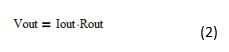Equation 3 sets the voltage regulation level: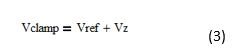As you can see in Figure 1, a Zener diode regulates the voltage in CV mode. Using a Zener as a voltage clamp will yield relatively poor voltage-accuracy performance because of the variation in Zener voltages from device to device. Two Zener diodes are sometimes used in series to prevent leakage current flowing from cathode to anode, which if present will cause errors in the current regulation loop.

The traditional method requires the use of a sense resistor in series with the output in order to sense current. As a result, resistive losses will impact efficiency; Equation 4 shows the losses in the sense resistor: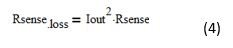Higher losses will increase the operating temperature and reduce system efficiency because the resistor will have all of the output current flowing through it. Cost also increases because low milliohm current-sense resistors are relatively expensive compared to a small signal resistor.

The common-mode voltage range of the amplifier needs to be rated to the maximum output voltage. A high output voltage might increase the cost of the amplifier. To help save costs, you could use a floating bias supply to reduce the common-mode voltage range requirement, but that will increase the component count.

The solution presented in Figure 1 has many disadvantages, including added design complexity, board real estate required, cost and impact on system efficiency.

A simple CC/CV method using the LM5117

The LM5117 is an emulated peak current-mode synchronous buck controller suitable for high-current, wide stepdown conversions. The major benefit of using the LM5117 in a CC/CV application is that it has a current monitor (CM) feature. The CM pin provides an accurate voltage that is proportional to the output current of the buck power stage. You can use the CM pin as your current loop feedback, saving the additional current-sense circuitry that the traditional method requires. The voltage present on the CM pin is accurate to ±2%, provided that the converter is set to forced pulsed width modulation (FPWM) or is in continuous conduction mode. Figure 2 shows a basic CC/CV regulator implementation using the LM5117.Figure 2: LM5117 CC/CV basic implementation

CC programming

Equation 5 describes the relationship between the CM voltage and Iout: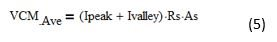Equation 6 simplifies Equation 5: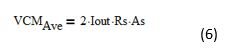As you can see, the CM pin enables you to omit the series power dissipative current-sense resistor at the output. Rs is the current-sense resistor of the power stage used to generate a ramp for the current-mode pulse-width modulation (PWM) loop. As is the current-sense amplifier gain of the LM5117, which has a typical value of As = 10.

For example, assume that Iout = 10A and Rs = 10mΩ. Using Equation 6: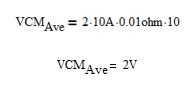Setting the resistor divider network from the CM pin to ground and connecting the divider node to the feedback pin sets the current regulation point. With 2V at the CM pin, selecting the proper resistor-divider ratio will set the current regulation level. To set the resistor-divider values for 10A current regulation, select RFBb = 10kΩ and calculate RFBt using Equation 7: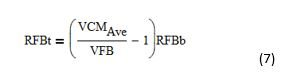Which yields an RFBt value of 15kΩ.

Remember to account for the reduction in As caused by placing a resistor from Rs and ground to Cs and Csg, respectively. Refer to the LM5117 data sheet for more details on how resistors in series with the current-sense pins affect the gain of the internal current-sense amplifier.

CV programming

CV programing is achieved by using an LMV431 as a voltage clamp.  Assume a voltage clamp level of 12V. The forward voltage drop Vfwd across BAT54 is 0.5V and the FB voltage of the LM5117 is 0.8V. The voltage clamp engages when the voltage across R1 is equal to the voltage calculated using Equation 8: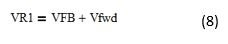Therefore, VR1 = 1.3V.

The voltage at the reference pin of the LMV431 will need to be a reference voltage above VR1. The LMV431 has a reference voltage of 1.24V, so the voltage at the reference pin of the LMV431 is equal to the voltage calculated using Equation 9: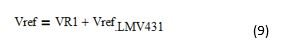Therefore, a Vref = 2.54V is required for the LMV431 to conduct current from its cathode to anode.

Select RBotVC = 10kΩ and calculate RTopVC using Equation 10: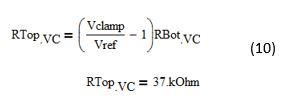Power design of the LM5117

The design approach for the power section of a CC/CV converter using the LM5117 is the same as it is for a basic buck converter. I suggest carrying out the design at the highest output power level (which is the highest output resistance) using either WEBENCH® Designer or the LM5117 Quick Start Calculator. You can also refer to the LM5117 data sheet for guidance on the design of the buck power stage.

Example schematic

Figure 3 shows a 30V-to-54V input, 27V-at-6A output CC/CV implementation using the LM5117.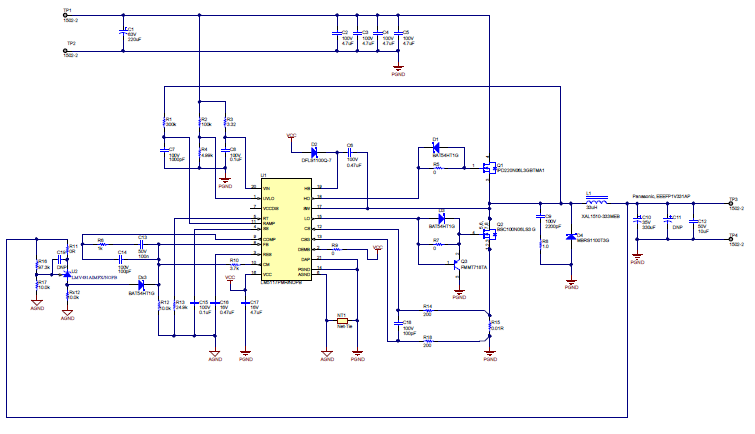Figure 3: A 30V-to-54V input, 27V-at-6A output CC/CV converter using the LM5117

Results

Figure 4 shows the efficiency results with increasing output resistance.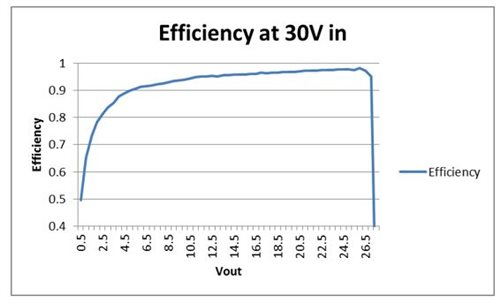Figure 4: Efficiency at 30Vin

Figure 5 shows load regulation and the voltage set point with increasing output resistance.Figure 6 shows the switch node (CH3), Vout ripple (CH1) and output current (CH4) at 30Vin, 25Vout at 6A.Figure 7 shows the load-transient performance Vout (CH1) and output current (CH4) when stepping a constant resistive load from 60Ω to 120Ω.Summary

The LM5117 configured as a CC/CV converter provides accurate current regulation, while offering many advantages over the traditional implementation. The design approach is relatively simple, and enables significant reductions in size, cost and power losses. Start a power supply design now with the LM5117 using WEBENCH® Designer.

• I need constant current = 15A at app 48V.

Will it work for the LM5145 for instance … if I use an amplified (ground-referenced) current-sense signal as "Vout"-feedback signal ?

(Btw, resolution of pictures does not allow reading the values of oscilloscope and of schematics.)

• Hello Marco,

By not having a current monitor pin you will have to implement an output current to feedback voltage conversion using an amplifier.  You can use the LM5145 and implement an amplifier scheme discussed in this article, as highlighted in the article you can use an amplifier with a high common voltage range or use a floatng supply, referenced to Vout.

Hope this helps?

David.

• Is there a higher resolution copy of Figure 3 (the schematic) that is readable ? And a BOM that matches Figure 3?

I plan to implement this onto  "AN-2103 LM25117 Evaluation Board SNVA466B" to give me a 4A, DC constant current source with the highest output voltage clamp possible with a 48VIN.

• Please see link below for app note, which includes a higher resolution image.

www.ti.com/.../snva829.pdf

Hope this helps?

David.

• Hi David,

a great article. I love the NSC spirit, please keep it..

In my opinion the circuit shown in Figure 2 will have quite big temperature drift because of the Vfd of the diode.  I suggest adding a second diode in series with the RbotVC. This would do the job (assuming same type, Vfd and temp. drift).

Regards, Jiri

• Thanks for your input Jiri,

Your temperature compensation idea is a good one and will work well minimize drift in voltage clamp over temperature.

David.

• Does it work on boost controller?

Thanks for sharing.

• Hello Troy,

The LM5117 is a buck controller and cannot be configured as a boost.  In general a CC/CV can be implement as a boost provided the condition of Vin<Vout is always met when controlling the output current.  If the condition of Vin>Vout occurs when trying to regulate the current, it will no longer work as a CC regulator.  You would need to implement a CC/CV for Vin<Vout  and Vin>Vout using a buck boost topology, using the LM5175.  In fact the LM5175 has an Iave function to do just that.

Hope this helps?

David.

• Hi David,

I mean figure 1.Can I place figure 1 with boost converter?

Troy

• Hello troy,

Understood, the answer I gave still applies to Figure 1.  Yes, you can implement, provided Vout, as it adjusts, as it controls Iout, is always greater than Vin.  If ever Vin>Vout, you will lose control of the current.

Hope this helps?

David.

• Hi David,

Troy

• Could you please explain step by step how to workout Equation 1 ? and what is the mathematical relationship between the current through the sense resistor and the current through RFB, how does the OPAMP controlles the transistor base current ?

• Hello,

It assumes the voltage at the input of the op amp [+ve/-ve]  this means the voltage across the Rin resistor is the same voltage across Rsense.  Also we assume the current in the collector of the pnp is the same as the emitter current in the pnp.

We can calculate the emitter current as V(Rin)/Rin = Iemitter and V(Rin) = Iout*Rsense

in order to be in current regulation the feedback voltage is set at the reference voltage of the controller being used (assume 0.8V).

We can therefore say

Iout*Rsense/Rin = Iemitter = Icollector

And Icollector *Rfb= Vfb

thererfore

(Iout*Rsense/(Rin))*Rfb =Vfb

Transposing for Iout will yield Equation 1.  Hope this helps?